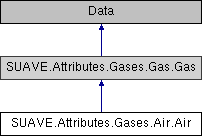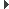SUAVE  2.5.2 An Aerospace Vehicle Environment for Designing Future AircraftSUAVE.Attributes.Gases.Air.Air Class Reference
Inheritance diagram for SUAVE.Attributes.Gases.Air.Air:## Public Member Functions

def __defaults__ (self)

def compute_density (self, T=300., p=101325.)

def compute_speed_of_sound (self, T=300., p=101325., variable_gamma=False)

def compute_cp (self, T=300., p=101325.)

def compute_gamma (self, T=300., p=101325.)

def compute_absolute_viscosity (self, T=300., p=101325.)

def compute_thermal_conductivity (self, T=300., p=101325.)

def compute_prandtl_number (self, T=300.)

## Public Attributes

tag

molecular_mass

gas_specific_constant

specific_heat_capacityPublic Attributes inherited from SUAVE.Attributes.Gases.Gas.Gas
tag

molecular_mass

gas_specific_constant

composition

## Detailed Description

```Holds constants and functions that compute gas properties for air.

Assumptions:
None

Source:
None
```

## ◆ __defaults__()

 def SUAVE.Attributes.Gases.Air.Air.__defaults__ ( self )
```This sets the default values.

Assumptions:
None

Source:
Values commonly available

Inputs:
None

Outputs:
None

Properties Used:
None
```

Reimplemented from SUAVE.Attributes.Gases.Gas.Gas.

## ◆ compute_absolute_viscosity()

 def SUAVE.Attributes.Gases.Air.Air.compute_absolute_viscosity ( self, T = `300.`, p = `101325.` )
```Compute the absolute (dynamic) viscosity

Assumptions:
Ideal gas

Source:
https://www.cfd-online.com/Wiki/Sutherland's_law

Inputs:
T                  [K]       - Temperature

Outputs:
absolute viscosity [kg/(m-s)]

Properties Used:
None
```

## ◆ compute_cp()

 def SUAVE.Attributes.Gases.Air.Air.compute_cp ( self, T = `300.`, p = `101325.` )
```Computes Cp by 3rd-order polynomial data fit:
cp(T) = c1*T^3 + c2*T^2 + c3*T + c4

Coefficients (with 95% confidence bounds):
c1 = -7.357e-007  (-9.947e-007, -4.766e-007)
c2 =    0.001307  (0.0009967, 0.001617)
c3 =     -0.5558  (-0.6688, -0.4429)
c4 =        1074  (1061, 1086)

Assumptions:
123 K < T < 673 K

Source:
Unknown, possibly Combustion Technologies for a Clean Environment
(Energy, Combustion and the Environment), Jun 15, 1995, Carvalhoc

Inputs:
T              [K]       - Temperature
p              [Pa]      - Pressure

Outputs:
cp             [J/kg-K]

Properties Used:
None
```

## ◆ compute_density()

 def SUAVE.Attributes.Gases.Air.Air.compute_density ( self, T = `300.`, p = `101325.` )
```Computes air density given temperature and pressure

Assumptions:
Ideal gas

Source:
Common equation

Inputs:
T         [K]  - Temperature
p         [Pa] - Pressure

Outputs:
density   [kg/m^3]

Properties Used:
self.gas_specific_constant
```

## ◆ compute_gamma()

 def SUAVE.Attributes.Gases.Air.Air.compute_gamma ( self, T = `300.`, p = `101325.` )
```Computes Cp by 3rd-order polynomial data fit:
gamma(T) = c1*T^3 + c2*T^2 + c3*T + c4

Coefficients (with 95% confidence bounds):
c1 =  1.629e-010  (1.486e-010, 1.773e-010)
c2 = -3.588e-007  (-3.901e-007, -3.274e-007)
c3 =   0.0001418  (0.0001221, 0.0001614)
c4 =       1.386  (1.382, 1.389)

Assumptions:
233 K < T < 1273 K

Source:
Unknown

Inputs:
T              [K]       - Temperature
p              [Pa]      - Pressure

Outputs:
g              [-]

Properties Used:
None
```

## ◆ compute_prandtl_number()

 def SUAVE.Attributes.Gases.Air.Air.compute_prandtl_number ( self, T = `300.` )
```Compute the prandtl number

Assumptions:

Source:
N/A

Inputs:
specific_heat_capacity [J/kgK]
absolute viscosity     [kg/(m-s)]
thermal conductivity   [W/(m-K)]

Outputs:
prandtl number         [unitless]

Properties Used:
None
```

## ◆ compute_speed_of_sound()

 def SUAVE.Attributes.Gases.Air.Air.compute_speed_of_sound ( self, T = `300.`, p = `101325.`, variable_gamma = `False` )
```Computes speed of sound given temperature and pressure

Assumptions:
Ideal gas with gamma = 1.4 if variable gamma is False

Source:
Common equation

Inputs:
T              [K]       - Temperature
p              [Pa]      - Pressure
variable_gamma <boolean> - Determines if gamma is computed

Outputs:
speed of sound [m/s]

Properties Used:
self.compute_gamma() (if variable gamma is True)
self.gas_specific_constant
```

## ◆ compute_thermal_conductivity()

 def SUAVE.Attributes.Gases.Air.Air.compute_thermal_conductivity ( self, T = `300.`, p = `101325.` )
```Compute the thermal conductivity

Assumptions:
Properties computed at 1 bar (14.5 psia)

Source:
https://www.engineeringtoolbox.com/air-properties-viscosity-conductivity-heat-capacity-d_1509.html

Inputs:
T                  [K]       - Temperature

Outputs:
thermal conductivity [W/(m-K)]

Properties Used:
None
```

The documentation for this class was generated from the following file:
• /Users/emiliobotero/Dropbox/SUAVE/SUAVE/trunk/SUAVE/Attributes/Gases/Air.py